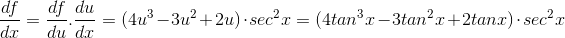# High School Math : Derivative Review

## Example Questions

### Example Question #1 : Derivatives

Find the second derivative of f(x).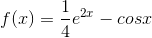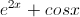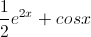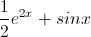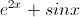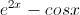Explanation:

First we should find the first derivative of. Remember the derivative of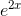is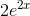and the derivative of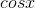is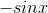: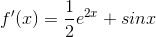The second derivative is just the derivative of the first derivative: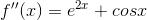### Example Question #4 : Understanding The Derivative Of Trigonometric Functions

Find the derivative of the function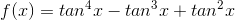.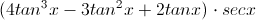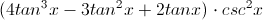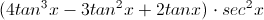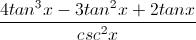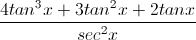Explanation:

We can use the Chain Rule:

Let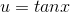, so that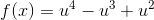.